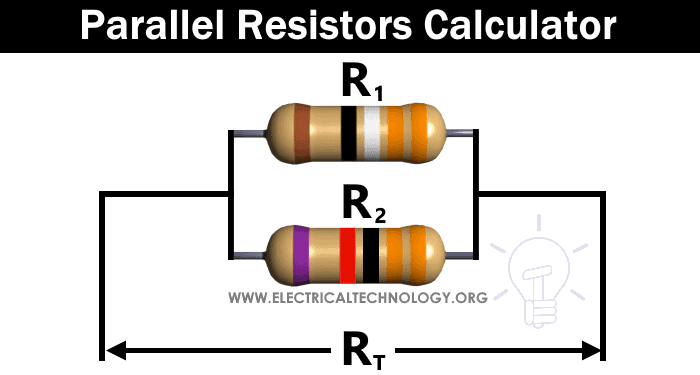# Parallel Resistors Calculator

## Resistance of Parallel Connected Resistors Calculator

### Formula & Equations for Parallel Resistors Calculator

Finding Total Resistance (RT) when R1 and R2 are given;

1/RT = 1/R1 + 1/R2

RT = R1||R2

RT = R1 x R/ (R1 + R2)

Finding R1 when RT and R2 given;

R1 = R2× RT / (R2 – RT)

Finding R2 when RT and R1 given;

R2 = R× R/ (R1 – RT)### Parallel Resistors Calculator

Enter any two values and Select Unit then Click on Calculate. Result will display the required Value.

Enter values:
Resistor R1:
Resistor R2:

Parallel Resistance RT:

Related Calculators: